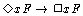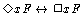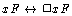# The Logic of EncodingExamples

For convenience, we reproduce the item Logic/Logic of Encoding from Principia Metaphysica:In this tutorial, we explain this axiom and then draw out some consequences.

## Explanation of the Axiom

This axiom asserts: if an object x possibly encodes property F, then x encodes F necessarily. In terms of our intuitions about possible worlds, then this tells us that if an object x encodes property F at any possible world, then it encodes F at every possible world. To appreciate the significance of this fact, let us contrast the situation with the logic of exemplification.

Intuitively, let us picture our object terms as naming or ranging over a fixed domain of objects and picture our relation terms as naming or ranging over a fixed domain of relations. Let us further imagine that there is a domain of possible worlds (our primitive notion `it is necessary that' is, intuitively, a universal quantifier that ranges over the domain of possible worlds). Since objects can exemplify properties and stand in relations at this world but not exemplify those same properties and stand in those same relations at every possible world, we may suppose that each n-place relation R in the domain of relations has an `exemplification extension' at each possible world. The exemplification extension of a relation R at a possible world w is a set of n-tuples, and each member of this set represents an ordered group of objects that exemplify or stand in the relation R at w. Now suppose that the variables x_1,...,x_n denote the objects o_1,...,o_n, respectively, and that the predicate `F' denotes the n-place relation R. Then we may say that the atomic sentence `Fx_1...x_n' is true at world w just in case the n-tuple <o_1,...,o_n> is an element of the exemplification extension of the relation R at w. In the 1-place case, where `x' denotes the object o and `F' denotes the property P, we may say that the atomic sentence `Fx' is true at world w if and only if o is a member of the exemplification extension of the property P.

Now since atomic encoding formulas are monadic (i.e., they always have a single object term to the left of a 1-place property term), we can describe their truth conditions as follows. Suppose that each 1-place property in the domain of relations receives, in addition to an exemplification extension, an `encoding extension'. Each object that is in the encoding extension of property P represents the fact that the object encodes P. But whereas the exemplification extension of a property or relation may vary from world to world, let us say that the encoding extension of a property does not vary from world to world. Indeed, the encoding extension of a property is not relativized to a world. We can, nevertheless, define world-relative truth conditions for atomic encoding formulas: when `x' denotes o and `F' denotes P, then the atomic formula `xF' is true at world w if and only if o is an element of the encoding extension of P.

Notice that, given these truth conditions, the following is a fact: if an atomic encoding formula is true at any world w, it is true at every world w. This fact, when expressed from the point of view of our language in which modality is primitive, is just our logical axiom for encoding, for that axiom asserts that if an atomic encoding formula is possibly true, it is necessarily true.

## Some Consequences:

Logical Theorem:This theorem asserts: an object possibly encodes a property if and only if it necessarily encodes that property . Clearly, the conditional in the left-to-right direction is true, since it is an instance of our logical axiom for encoding. The conditional in the right-to-left direction is true because if it is necessary that x encodes F, then it is true simpliciter that x encodes F, and so it is possibly true that x encodes F.

Logical Theorem:This theorem tells us that any property in fact encoded by an object is a property that is essentially encoded by that object, and vice versa. Abstract objects are individuated by the properties they encode. These properties are even more essential to their identity than are the properties they may necessarily exemplify. For example, the property being a detective is a constitutive property of Sherlock Holmes. This is a property Holmes encodes. It is property we use to specify which abstract object Holmes is. By contrast, the property of not being a building is a property that Holmes necessarily exemplifies. He shares this property with all other abstract objects. Though Holmes exemplifies this property necessarily, it is not one of the properties by which we individuate Holmes.